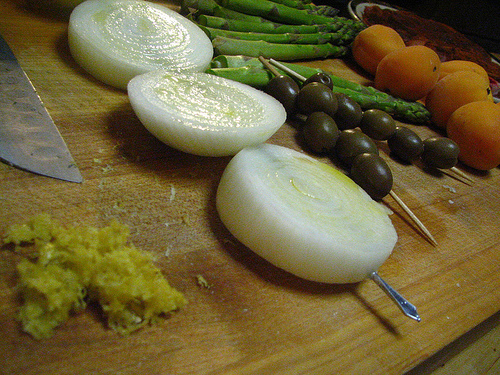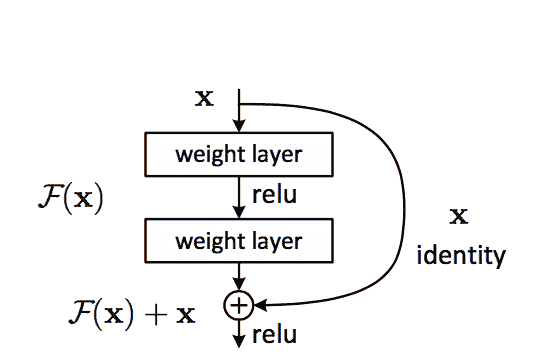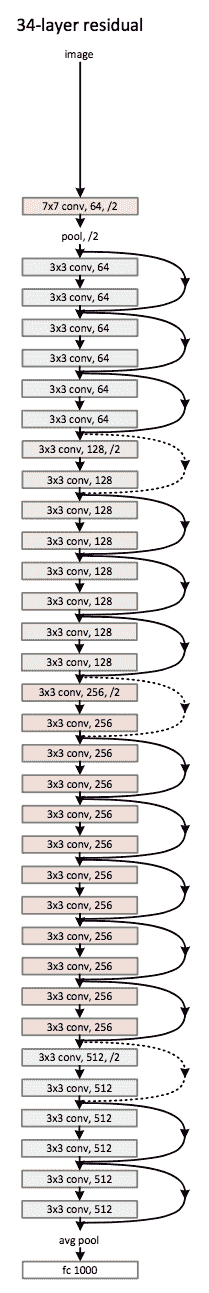# Deep Network Models

Constructing and training your own ConvNet from scratch can be Hard and a long task.

A common trick used in Deep Learning is to use a pre-trained model and finetune it to the specific data it will be used for.

## Famous Models with Keras

This notebook contains code and reference for the following Keras models (gathered from https://github.com/fchollet/keras/tree/master/keras/applications)

• VGG16
• VGG19
• ResNet50
• Inception v3
• Xception
• ... more to come

All architectures are compatible with both TensorFlow and Theano, and upon instantiation the models will be built according to the image dimension ordering set in your Keras configuration file at `~/.keras/keras.json`.

For instance, if you have set `image_data_format="channels_last"`, then any model loaded from this repository will get built according to the TensorFlow dimension ordering convention, "Width-Height-Depth".

# VGG16# VGG19# `keras.applications`

``````from keras.applications import VGG16
from keras.applications.imagenet_utils import preprocess_input, decode_predictions
import os
``````
``````Using TensorFlow backend.
``````
``````vgg16 = VGG16(include_top=True, weights='imagenet')
vgg16.summary()
``````
``````_________________________________________________________________
Layer (type)                 Output Shape              Param #
=================================================================
input_1 (InputLayer)         (None, 224, 224, 3)       0
_________________________________________________________________
block1_conv1 (Conv2D)        (None, 224, 224, 64)      1792
_________________________________________________________________
block1_conv2 (Conv2D)        (None, 224, 224, 64)      36928
_________________________________________________________________
block1_pool (MaxPooling2D)   (None, 112, 112, 64)      0
_________________________________________________________________
block2_conv1 (Conv2D)        (None, 112, 112, 128)     73856
_________________________________________________________________
block2_conv2 (Conv2D)        (None, 112, 112, 128)     147584
_________________________________________________________________
block2_pool (MaxPooling2D)   (None, 56, 56, 128)       0
_________________________________________________________________
block3_conv1 (Conv2D)        (None, 56, 56, 256)       295168
_________________________________________________________________
block3_conv2 (Conv2D)        (None, 56, 56, 256)       590080
_________________________________________________________________
block3_conv3 (Conv2D)        (None, 56, 56, 256)       590080
_________________________________________________________________
block3_pool (MaxPooling2D)   (None, 28, 28, 256)       0
_________________________________________________________________
block4_conv1 (Conv2D)        (None, 28, 28, 512)       1180160
_________________________________________________________________
block4_conv2 (Conv2D)        (None, 28, 28, 512)       2359808
_________________________________________________________________
block4_conv3 (Conv2D)        (None, 28, 28, 512)       2359808
_________________________________________________________________
block4_pool (MaxPooling2D)   (None, 14, 14, 512)       0
_________________________________________________________________
block5_conv1 (Conv2D)        (None, 14, 14, 512)       2359808
_________________________________________________________________
block5_conv2 (Conv2D)        (None, 14, 14, 512)       2359808
_________________________________________________________________
block5_conv3 (Conv2D)        (None, 14, 14, 512)       2359808
_________________________________________________________________
block5_pool (MaxPooling2D)   (None, 7, 7, 512)         0
_________________________________________________________________
flatten (Flatten)            (None, 25088)             0
_________________________________________________________________
fc1 (Dense)                  (None, 4096)              102764544
_________________________________________________________________
fc2 (Dense)                  (None, 4096)              16781312
_________________________________________________________________
predictions (Dense)          (None, 1000)              4097000
=================================================================
Total params: 138,357,544
Trainable params: 138,357,544
Non-trainable params: 0
_________________________________________________________________
``````

If you're wondering where this `HDF5` files with weights is stored, please take a look at `~/.keras/models/`

#### HandsOn VGG16 - Pre-trained Weights

``````IMAGENET_FOLDER = 'img/imagenet'  #in the repo
``````
``````!ls img/imagenet
``````
``````apricot_565.jpeg  apricot_787.jpeg    strawberry_1174.jpeg

apricot_696.jpeg  strawberry_1157.jpeg    strawberry_1189.jpeg
````````````from keras.preprocessing import image
import numpy as np

img_path = os.path.join(IMAGENET_FOLDER, 'strawberry_1157.jpeg')
x = image.img_to_array(img)
x = np.expand_dims(x, axis=0)
x = preprocess_input(x)
print('Input image shape:', x.shape)

preds = vgg16.predict(x)
print('Predicted:', decode_predictions(preds))
``````
``````Input image shape: (1, 224, 224, 3)
Predicted: [[('n07745940', 'strawberry', 0.98570204), ('n07836838', 'chocolate_sauce', 0.005128039), ('n04332243', 'strainer', 0.003665844), ('n07614500', 'ice_cream', 0.0021996102), ('n04476259', 'tray', 0.0011693746)]]
````````````img_path = os.path.join(IMAGENET_FOLDER, 'apricot_696.jpeg')
x = image.img_to_array(img)
x = np.expand_dims(x, axis=0)
x = preprocess_input(x)
print('Input image shape:', x.shape)

preds = vgg16.predict(x)
print('Predicted:', decode_predictions(preds))
``````
``````Input image shape: (1, 224, 224, 3)
Predicted: [[('n07747607', 'orange', 0.84150302), ('n07749582', 'lemon', 0.053847123), ('n07717556', 'butternut_squash', 0.017796788), ('n03937543', 'pill_bottle', 0.015318954), ('n07720875', 'bell_pepper', 0.0083615109)]]
````````````img_path = os.path.join(IMAGENET_FOLDER, 'apricot_565.jpeg')
x = image.img_to_array(img)
x = np.expand_dims(x, axis=0)
x = preprocess_input(x)
print('Input image shape:', x.shape)

preds = vgg16.predict(x)
print('Predicted:', decode_predictions(preds))
``````
``````Input image shape: (1, 224, 224, 3)
Predicted: [[('n07718472', 'cucumber', 0.37647018), ('n07716358', 'zucchini', 0.25893891), ('n07711569', 'mashed_potato', 0.049320061), ('n07716906', 'spaghetti_squash', 0.033613835), ('n12144580', 'corn', 0.031451162)]]
``````

# Hands On:

### Try to do the same with VGG19 Model

``````# from keras.applications import VGG19

# - Visualise Summary
# - Infer classes using VGG19 predictions
``````
``````# [your code here]
``````

# Residual Networks## ResNet 50``````from keras.applications import ResNet50
``````

A ResNet is composed by two main blocks: Identity Block and the ConvBlock.

• IdentityBlock is the block that has no conv layer at shortcut
• ConvBlock is the block that has a conv layer at shortcut
``````from keras.applications.resnet50 import identity_block, conv_block
``````
``````identity_block??
``````
``````conv_block??
``````

# Visualising Convolutional Filters of a CNN

``````import numpy as np
import time
from keras.applications import vgg16
from keras import backend as K
``````
``````from matplotlib import pyplot as plt

%matplotlib inline
``````
``````# dimensions of the generated pictures for each filter.
IMG_WIDTH = 224
IMG_HEIGHT = 224
``````
``````from keras.applications import vgg16

# build the VGG16 network with ImageNet weights
vgg16 = vgg16.VGG16(weights='imagenet', include_top=False)
``````
``````Model loaded.
``````
``````vgg16.summary()
``````
``````_________________________________________________________________
Layer (type)                 Output Shape              Param #
=================================================================
input_2 (InputLayer)         (None, None, None, 3)     0
_________________________________________________________________
block1_conv1 (Conv2D)        (None, None, None, 64)    1792
_________________________________________________________________
block1_conv2 (Conv2D)        (None, None, None, 64)    36928
_________________________________________________________________
block1_pool (MaxPooling2D)   (None, None, None, 64)    0
_________________________________________________________________
block2_conv1 (Conv2D)        (None, None, None, 128)   73856
_________________________________________________________________
block2_conv2 (Conv2D)        (None, None, None, 128)   147584
_________________________________________________________________
block2_pool (MaxPooling2D)   (None, None, None, 128)   0
_________________________________________________________________
block3_conv1 (Conv2D)        (None, None, None, 256)   295168
_________________________________________________________________
block3_conv2 (Conv2D)        (None, None, None, 256)   590080
_________________________________________________________________
block3_conv3 (Conv2D)        (None, None, None, 256)   590080
_________________________________________________________________
block3_pool (MaxPooling2D)   (None, None, None, 256)   0
_________________________________________________________________
block4_conv1 (Conv2D)        (None, None, None, 512)   1180160
_________________________________________________________________
block4_conv2 (Conv2D)        (None, None, None, 512)   2359808
_________________________________________________________________
block4_conv3 (Conv2D)        (None, None, None, 512)   2359808
_________________________________________________________________
block4_pool (MaxPooling2D)   (None, None, None, 512)   0
_________________________________________________________________
block5_conv1 (Conv2D)        (None, None, None, 512)   2359808
_________________________________________________________________
block5_conv2 (Conv2D)        (None, None, None, 512)   2359808
_________________________________________________________________
block5_conv3 (Conv2D)        (None, None, None, 512)   2359808
_________________________________________________________________
block5_pool (MaxPooling2D)   (None, None, None, 512)   0
=================================================================
Total params: 14,714,688
Trainable params: 14,714,688
Non-trainable params: 0
_________________________________________________________________
``````
``````from collections import OrderedDict
layer_dict = OrderedDict()
# get the symbolic outputs of each "key" layer (we gave them unique names).
for layer in vgg16.layers[1:]:
layer_dict[layer.name] = layer
``````

#### Test Image

``````img_path = os.path.join(IMAGENET_FOLDER, 'strawberry_1157.jpeg')
plt.imshow(img)
``````
``````<matplotlib.image.AxesImage at 0x7f896378cda0>
````````````input_img_data = image.img_to_array(img)
# input_img_data /= 255
plt.imshow(input_img_data)
``````
``````<matplotlib.image.AxesImage at 0x7f89636cd240>
````````````input_img_data = np.expand_dims(input_img_data, axis=0)
print('Input image shape:', input_img_data.shape)
``````
``````Input image shape: (1, 224, 224, 3)
``````

### Visualising Image throught the layers

``````## Recall the function defined in notebook on hidden features (2.1 Hidden Layer Repr. and Embeddings)

def get_activations(model, layer, input_img_data):
activations_f = K.function([model.layers.input, K.learning_phase()], [layer.output,])
activations = activations_f((input_img_data, False))
return activations
``````
``````layer_name = 'block1_conv2'
layer = layer_dict[layer_name]
activations = get_activations(vgg16, layer, input_img_data)
``````
``````print(len(activations))
activation = activations
activation.shape
``````
``````1

(1, 224, 224, 64)
``````
``````layer.filters  # no. of filters in the selected conv block
``````
``````64
``````
``````activated_img = activation
n = 8
fig = plt.figure(figsize=(20, 20))
for i in range(n):
for j in range(n):
idx = (n*i)+j
ax.imshow(activated_img[:,:,idx])
````````````conv_img_mean = np.mean(activated_img, axis=2)
``````
``````conv_img_mean.shape
``````
``````(224, 224)
``````
``````plt.imshow(conv_img_mean)
``````
``````<matplotlib.image.AxesImage at 0x7f895e8be668>
``````#### Now visualise the first 64 filters of the `block5_conv2` layer

``````layer_name = 'block5_conv2'
layer = layer_dict[layer_name]
activations = get_activations(vgg16, layer, input_img_data)
activated_img = activations  #  -> first (and only) activation, first (and only) sample in batch
n = 8
fig = plt.figure(figsize=(20, 20))
for i in range(n):
for j in range(n):
idx = (n*i)+j
ax.imshow(activated_img[:,:,idx])
``````## How Convnet see the world

#### Specify Percentage of Filters to scan

In this example, we'll still using VGG16 as the reference model.

Of course, the same code applies to different CNN models, with appriopriate changes in layers references/names.

Please note that VGG16 includes a variable number of convolutional filters, depending on the particular layer(s) selected for processing.

Processing all the convolutional filters may be a high intensive computation and time consuming and largely depending on the number of parameters for the layer.

On my hardwarde (1 Tesla K80 GPU on Azure Cloud) processing one single filter takes almost ~.5 secs. (on avg)

So, it would take ~256 secs (e.g. for `block5_conv1`) \$\mapsto\$ ~4mins (for one single layer name)

``````# utility function to convert a tensor into a valid image

def deprocess_image(x):
# normalize tensor: center on 0., ensure std is 0.1
x -= x.mean()
x /= (x.std() + 1e-5)
x *= 0.1

# clip to [0, 1]
x += 0.5
x = np.clip(x, 0, 1)

# convert to RGB array
x *= 255
if K.image_data_format() == 'channels_first':
x = x.transpose((1, 2, 0))
x = np.clip(x, 0, 255).astype('uint8')
return x
``````
``````# dimensions of the generated pictures for each filter.
img_width = 224
img_height = 224

def collect_filters(input_tensor, output_tensor, filters):
kept_filters = []
start_time = time.time()
for filter_index in range(0, filters):
if filter_index % 10 == 0:
print('\t Processing filter {}'.format(filter_index))

# we build a loss function that maximizes the activation
# of the nth filter of the layer considered
if K.image_data_format() == 'channels_first':
loss = K.mean(output_tensor[:, filter_index, :, :])
else:
loss = K.mean(output_tensor[:, :, :, filter_index])

# we compute the gradient of the input picture wrt this loss
# normalization trick: we normalize the gradient by its L2 norm
# this function returns the loss and grads given the input picture

# step size for gradient ascent
step = 1.

# we start from a gray image with some random noise
if K.image_data_format() == 'channels_first':
img_data = np.random.random((1, 3, img_width, img_height))
else:
img_data = np.random.random((1, img_width, img_height, 3))

img_data = (img_data - 0.5) * 20 + 128

# we run gradient ascent for 20 steps
for i in range(20):
if loss_value <= 0.:
# some filters get stuck to 0, we can skip them
break

# decode the resulting input image
if loss_value > 0:
img_deproc = deprocess_image(img_data)
kept_filters.append((img_deproc, loss_value))

end_time = time.time()
print('\t Time required to process {} filters: {}'.format(filters, (end_time - start_time)))

return kept_filters
``````
``````# this is the placeholder for the input images
input_t = vgg16.input

def generate_stiched_filters(layer, nb_filters):
layer_name = layer.name
print('Processing {} Layer'.format(layer_name))

# Processing filters of current layer
layer_output = layer.output
kept_filters = collect_filters(input_t, layer_output, nb_filters)

print('Filter collection: completed!')
# we will stich the best sqrt(filters_to_scan) filters put on a n x n grid.
limit = min(nb_filters, len(kept_filters))
n = np.floor(np.sqrt(limit)).astype(np.int)

# the filters that have the highest loss are assumed to be better-looking.
# we will only keep the top 64 filters.
kept_filters.sort(key=lambda x: x, reverse=True)
kept_filters = kept_filters[:n * n]

# build a black picture with enough space for
margin = 5
width = n * img_width + (n - 1) * margin
height = n * img_height + (n - 1) * margin
stitched_filters = np.zeros((width, height, 3))

# fill the picture with our saved filters
for i in range(n):
for j in range(n):
img, loss = kept_filters[i * n + j]
stitched_filters[(img_width + margin) * i: (img_width + margin) * i + img_width,
(img_height + margin) * j: (img_height + margin) * j + img_height, :] = img
return stitched_filters
``````
``````layer = layer_dict['block1_conv2']  # 64 filters
stitched_filters = generate_stiched_filters(layer, layer.filters)
plt.figure(figsize=(10,10))
plt.imshow(stitched_filters)
``````
``````Processing block1_conv2 Layer
Processing filter 0
Processing filter 10
Processing filter 20
Processing filter 30
Processing filter 40
Processing filter 50
Processing filter 60
Time required to process 64 filters: 22.710692167282104
Filter collection: completed!

<matplotlib.image.AxesImage at 0x7f895737ca90>
````````````layer = layer_dict['block5_conv1']  # 512 filters in total
stitched_filters = generate_stiched_filters(layer, 64)
plt.figure(figsize=(10,10))
plt.imshow(stitched_filters)
``````
``````Processing block5_conv1 Layer
Processing filter 0
Processing filter 10
Processing filter 20
Processing filter 30
Processing filter 40
Processing filter 50
Processing filter 60
Time required to process 64 filters: 101.60693192481995
Filter collection: completed!

<matplotlib.image.AxesImage at 0x7f884e7d7c50>
``````